Open in App
Not now

# ROWS and COLUMNS Functions in Excel With Examples

• Difficulty Level : Basic
• Last Updated : 01 Jun, 2021

In this article, we are going to see the details about Excel ROW, ROWS, COLUMN, COLUMNS functions, and their usages.

## ROW Function

The ROW( ) function basically returns the row number for a reference.

Syntax:

`=ROW([reference])`

Example :

```=ROW(G100); Returns 100 as output
as G100 is in the 100th row in the Excel sheet```

If we write no argument inside the ROW( ) it will return the row number of the cell in which we have written the formula.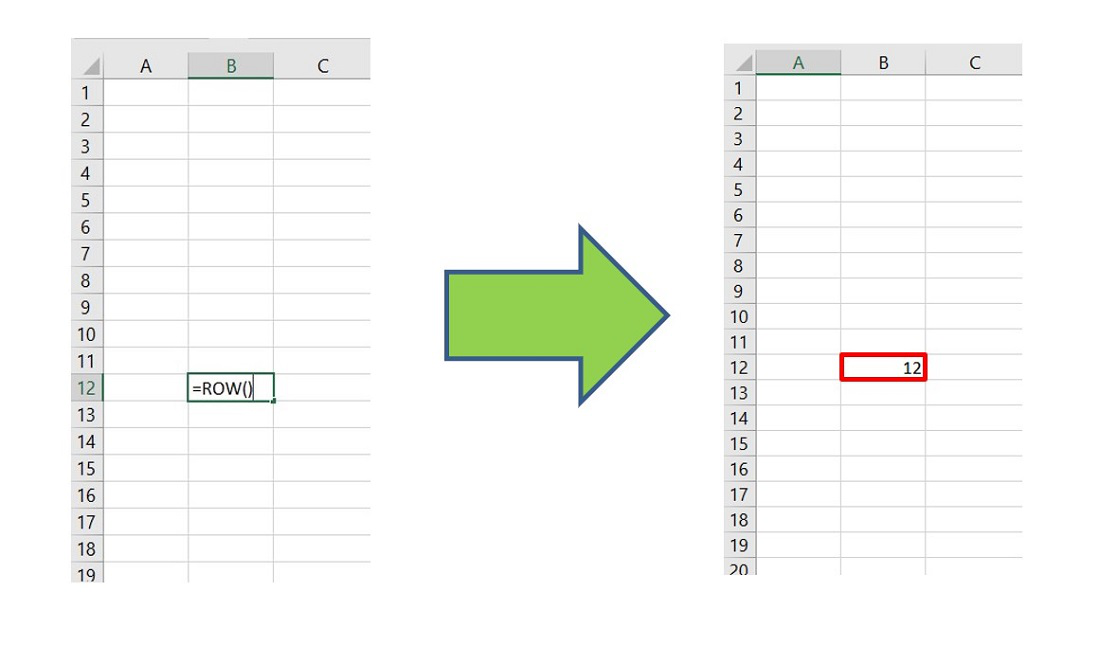Here the following returns are achieved:

```=ROW(A67) // Returns 67
=ROW(B4:H7) // Returns 4```

## ROWS Function

It is the extended version of the ROW function. ROWS function is used to return the number of rows in a cell range.

Syntax:

`=ROWS([array])`

Example :

`=ROWS(A2:A11) // Returns 10 as there are ten rows`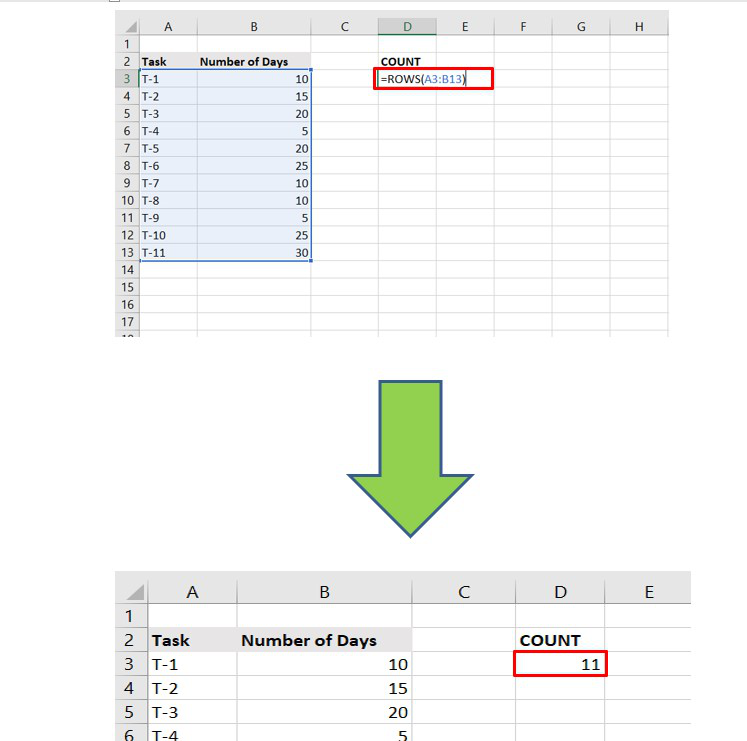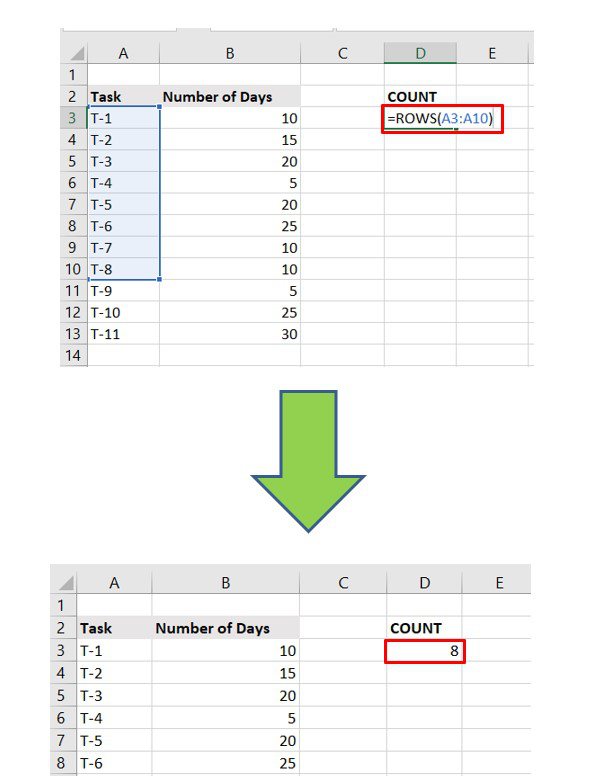It is important to note that writing the column name is trivial here. We can also write :

`=ROWS(2:13) // Returns 12`

## COLUMN Function

The column function usually returns the column number of a reference. There are 16,384 columns in Excel from the 2007 version. It returns the value 1 to 16,384.

Syntax:

`=COLUMN([reference])`

Example :

```=COLUMN(E20) // Returns 5 as output
as E is the fifth column in the Excel sheet```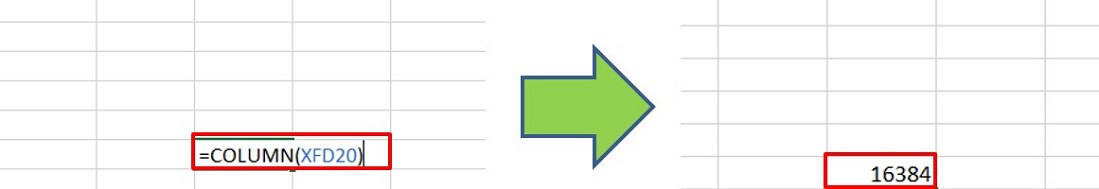Last Column of Excel

Similar to the ROW function, the argument is not mandatory. If we don’t write any argument, it will return the index of the column in which the formula is written.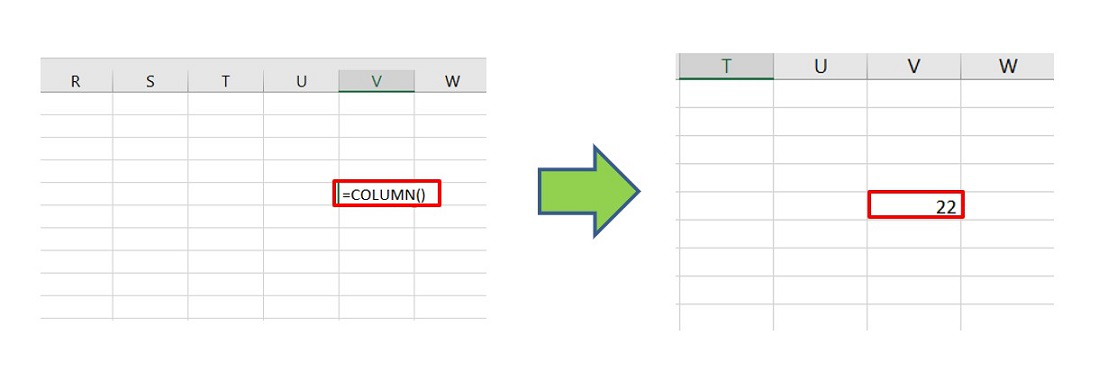## COLUMNS Function

It is the extended version of the COLUMN( ). It returns the number of columns for a given cell range.

Syntax:

`=COLUMNS([array])`

Example :

```=COLUMNS(B12:E12) // Returns 4 as output as
there are 4 columns in the given range```

It is important to note that writing the row number is trivial here. We can also write :

`=COLUMNS(B:E) // Returns 4`

## ROWS And COLUMNS Functions in Array Formulas

We can use the ROWS( ) and COLUMNS( ) in array formulas using SMALL( ), LARGE( ), VLOOKUP( ) functions in Excel.

Example :

The smallest value is returned to the provided cell range.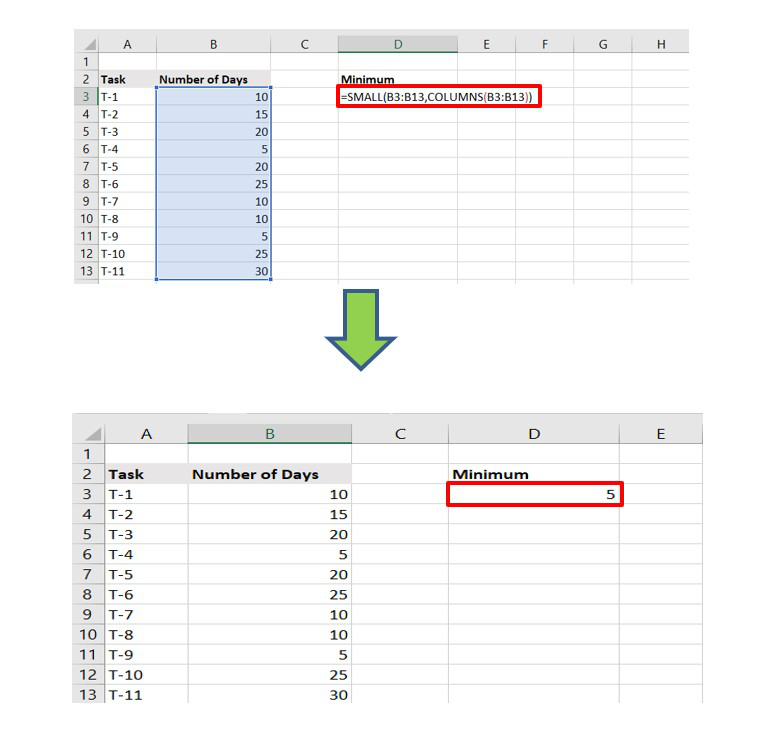The largest value is returned to the provided cell range.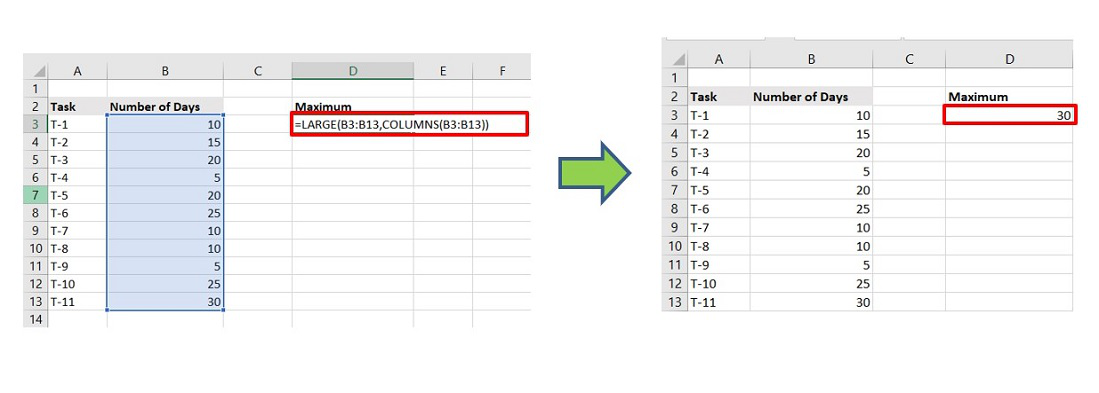Say, we need to find the number of days needed to complete Task number 5.

The steps are :

Step 1: Choose any cell and write the name of the Task.

Step 2:  Beside that cell write the “Formula” as shown below to get the “Number of Days” need :

=VLOOKUP(\$F\$3,\$A\$3:\$B\$13,COLUMNS(\$A\$3:\$B\$13),0)

F3 : Reference of the Task T-5

A3:B13 : Cell range of the entire table for VLOOKUP to search

Step 3: Click Enter.

Now, it can be observed it returns 20 as output which is perfect as per the data set.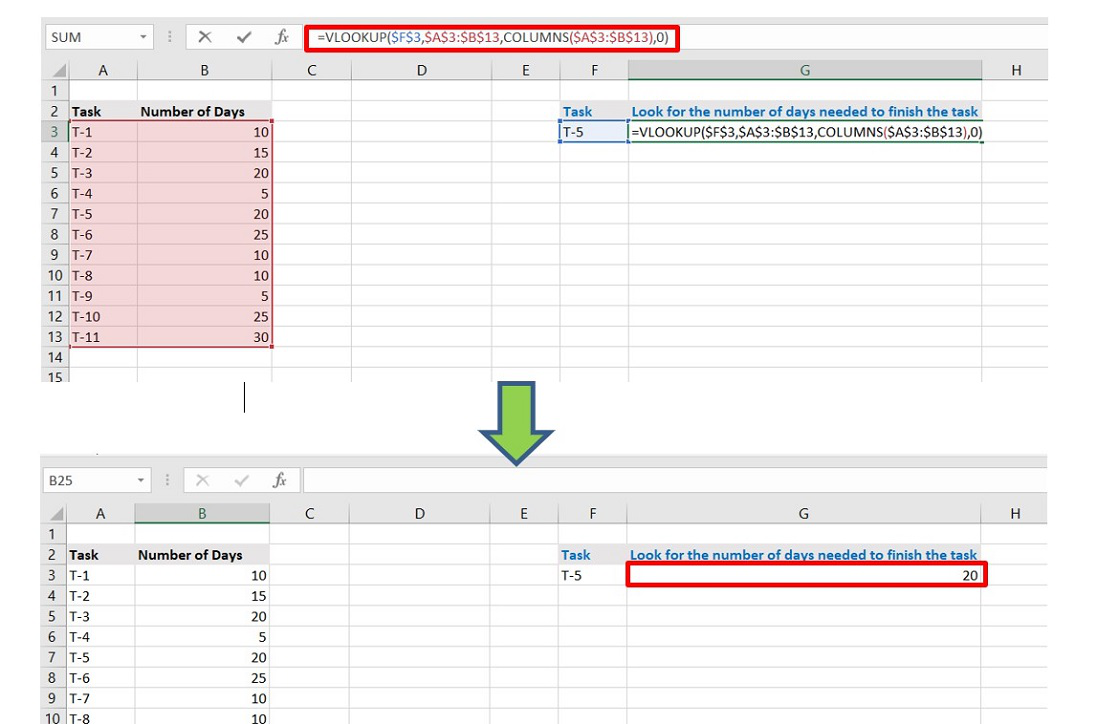My Personal Notes arrow_drop_up